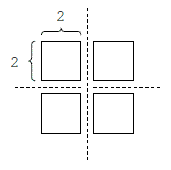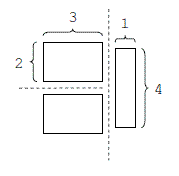문제1501--Cake Cutting

### 1501: Cake Cutting

실행시간 제한: 1 Sec  메모리사용 제한: 64 MB
제출: 117  통과: 60
[제출] [채점기록] [묻고답하기]

#### 문제 설명

You are given a rectangular cake of integral dimensions w × h. Your goal is to divide this cake into m rectangular pieces of integral dimensions such that the area of the largest piece is minimal. Each cut must be a straight line parallel to one of the sides of the original cake and must divide a piece of cake into two new pieces of positive area. Note that since a cut divides only a single piece, exactly m − 1 cuts are needed.

If w = 4, h = 4, and m = 4, then the following cuts minimize the area of the largest piece:However, if w = 4, h = 4, and m = 3, then the following cuts are optimal:#### 입력 설명

The input test file will contain multiple test cases, each of which consists of three integers w, h, m separated by a single space, with 1 ≤ w, h, m ≤ 20 and mwh. The end-of-file is marked by a test case with w = h = m = 0 and should not be processed.

#### 출력 설명

For each test case, write a single line with a positive integer indicating the area of the largest piece.

#### 입력 예시 Copy

4 4 4
4 4 3
0 0 0

#### 출력 예시 Copy

4
6